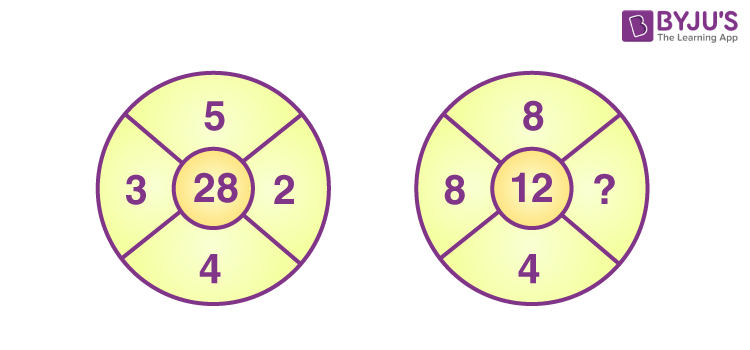# Find the missing number in the figure which follows the same patternIn the figure,

28 = ( 3 + 4 ) × (5 – 3 + 2)

Therefore, for the second figure

12 = (8 + 4) × (8 – 8 + 1)

The missing number is 1(0)(0)